Alkane Bonding, Intermolecular Forces, and Functional Groups

Alkane Bonding, Intermolecular Forces, and Functional Groups

Key Terms

• Sigma (σ) bond
• sp3 hybrid orbital
• Bonding orbital
• Antibonding orbital
• van der Waals forces
• Functional group

Objectives

• Understand how orbital hybridization affects carbon's bonding characteristics
• Recognize a sigma (σ) bond
• Apply van der Waals forces to basic alkane characteristics, like boiling point
• Distinguish between alkanes and cycloalkanes and organic molecules with functional groups

Alkane Bonding

Note that electrons seem to behave more like waves than like particles. So what is happening between two carbon atoms or a carbon atom and a hydrogen atom to cause them to "stick" together?

Because we cannot see atoms, we cannot be entirely sure. Nevertheless, a qualitative application of quantum theory can help explain how bonding occurs. If electrons act like waves in orbitals, then, presumably, those orbitals can overlap in such a manner that the waves constructively interfere (as opposed to destructive interference). This constructive interference occurs between the nuclei of the atoms as they approach one another, and it is related to the fact that the negatively charged electrons are attracted to the area between the positively charged nuclei owing to the electrostatic force. In the case of two hydrogen atoms, the spherical 1s orbitals overlap to form an ellipsoid-like orbital, as shown below (drawing not to scale), that represents the orbital of the bonded molecule H2.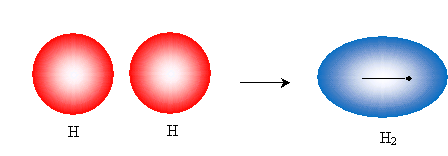This picture is simple enough: each hydrogen has a half-filled 1s orbital, and the overlapping orbitals yield a single full 1s orbital (i.e., a full valence shell) shared by both atoms. The hydrogen nuclei approach until the constructive interference of the electron wave functions is balanced by the repulsion of the positively charged nuclei (as well as the repulsion of the electrons, to some extent). At this point, the energy of the two-hydrogen "system" is minimized, and the hydrogen molecule is stable. The distance between the nuclei (the bond length) in this case is 74 picometers, or 0.000000000074 meters. This type of bond, where the orbitals overlap along the line formed by the two nuclei is called a sigma (σ) bond. Interestingly, though, this simple orbital view doesn't suffice for carbon.

Carbon has four valence electrons: two in its 2s orbital and two in its 2p orbital (one in each of two directional 2p orbitals, to be precise-2px and 2py, for instance). Following the above model, carbon would then only be able to form two covalent bonds, thereby filling up the two half-full directional 2p orbitals. But, as we have seen, carbon forms four covalent bonds to fill up its valence shell. One theory is that in its molecular form (that is, when bonded to other atoms), the 2s and 2p orbitals mix to form hybrid orbitals-in this case, sp3 hybrid orbitals. This type of hybrid orbital is one-quarter s-like and three-quarters p-like (because these orbitals are a mix of one s orbital and three p orbitals). A rough sketch of one of the sp3 orbitals is shown below.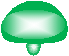Drawing the four sp3 orbitals as slightly elongated single lobes (emphasizing the major portion of the orbital drawn above) for illustration purposes, a carbon atom would "look" something like the tetrahedral sketch below.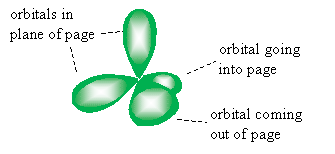A carbon-hydrogen covalent bond forms when the 1s orbital of a hydrogen atom overlaps one of the hybrid orbitals (in the case of an alkane, an sp3 orbital). Note that we represent electrons as arrows for the purposes of this illustration: these arrows are oriented either up or down to indicate their relative spins (or spin quantum numbers). Recall that for two electrons to exist in the same orbital, they must have different spin quantum numbers.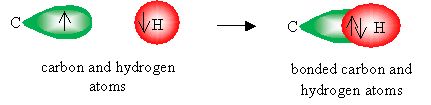Another view of bonding is that free atoms have atomic orbitals, but atoms in molecules have molecular orbitals. The total number of molecular orbitals is the sum of the atomic orbitals, and these molecular orbitals are divided into lower-energy (and thus more stable) bonding orbitals and higher-energy (and thus less stable) antibonding orbitals. Consider, again, the simple case of two hydrogen atoms. Each atom has one atom in its atomic orbital, but when combined to form H2, these atoms form two molecular orbitals. Following the sigma bond notation, the bonding orbital is labeled σ, whereas the antibonding orbital is labeled σ*.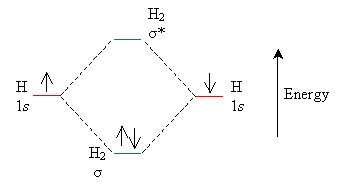This molecular orbital model is complementary-rather than contradictory-to the hybrid orbital model ("valence bond model") discussed previously. Each can be helpful in its own way in the study of organic chemistry.

Intermolecular Forces

At first glance, alkanes-and other organic molecules with a balance of positive and negative charges-would seem to tend to "ignore" each other, since they are net neutral molecules. But electrons are not always evenly distributed around nuclei, and as such, molecules may actually be electric dipoles (that is, they may have a dipole moment). Dipoles, even though they may, as a whole, be charge neutral, nonetheless exhibit a weak electric field that can influence other charges, dipoles, or even neutral molecules with no inherent dipole moment. Furthermore, the mobility of electrons can actually cause two neutral molecules with no inherent dipole moments to induce such dipole moments in each other (this electron mobility also explains why two neutral hydrogen atoms can attract each other). These induced dipoles may then weakly attract one another. The forces of attraction between neutral molecules owing to dipole moments or induced dipole moments are called van der Waals forces. To these forces, some of the characteristics of organic molecules, such as alkanes, can be attributed.

Consider, for instance, the boiling point of simple alkanes like methane, ethane, and propane. Because these molecules do not exhibit a significant dipole moment, the only van der Waals force that exists between them is due to induced dipoles. Thus, two methane molecules might form mutually induced dipoles, causing a weak attraction between them.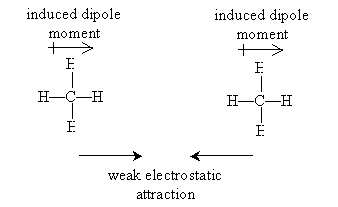At sufficiently a low temperature (i.e., the boiling point), the van der Waals force between the molecules is sufficient to cause them to "stick" together in a somewhat orderly manner-in other words, the substance goes from a gas (virtually no order owing to high energy of motion) to a liquid (slight order owing to lower energy of motion). Larger alkanes-in particular, those that are longer, less branched chains-have more possible locations in which dipole moments can be induced, meaning that the van der Waals forces between these larger molecules can accumulate. The result is that more energy of motion is required to break these forces, and therefore, the boiling point is higher.For example, the boiling point of methane is -160°C, ethane is -89°C, and propane is -42°C.

Combustion

You've probably heard of propane: a gas that is burned for heat in homes and cooking appliances, for example. Alkanes (and other hydrocarbons) burn (combust) rather easily, producing carbon dioxide, water, and heat when burned in the presence of oxygen. (Combustion is a type of oxidation-reduction reaction.) For instance, the following is a balanced chemical equation for the combustion of n-hexane:

2C6H14 + 19O2 → 12CO2 + 14H2O + Heat

In the case of n-hexane, combustion yields 4,163kJ/mol of heat.

Practice Problem: Write a balanced chemical equation for the combustion of ethylcyclohexane in pure oxygen.

Solution: First, we must determine a molecular formula for ethylcyclohexane. By following the IUPAC rules "in reverse," we obtain the molecule shown below. We can then count hydrogen and carbon atoms to get the molecular formula.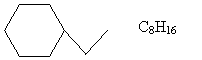Now, write the chemical equation, making sure that the numbers of each element are the same on both sides.

C8H16 + 12O2 → 8CO2 + 8H2O

Functional Groups

We have reviewed alkanes, the simplest hydrocarbons. But what about carbon compounds that have multiple bonds between carbon atoms or that incorporate atoms other than carbon and hydrogen? These multiple bonds and different atoms are largely responsible for the varying properties (reactivity) of different organic molecules. Specific groups of atoms that give organic molecules their reactive characteristics are called functional groups.

Alkanes are generally viewed as fundamental molecules that do not have functional groups. But when a double or triple bond between carbon atoms is added, or some atom or group of atoms, then functional groups are present. The following molecules each include a functional group (shown in red).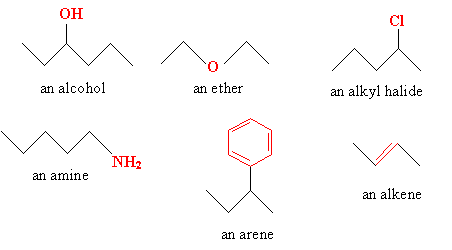Of course, a molecule may incorporate a number of functional groups, and the above are just a few examples. As with alkanes, organic molecules have specific rules for nomenclature that help to identify a molecule accurately. In some cases, these rules can yield long and highly complicated names; sometimes, it's easier just to use the common name for a substance, as with the molecule below.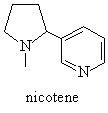Explore